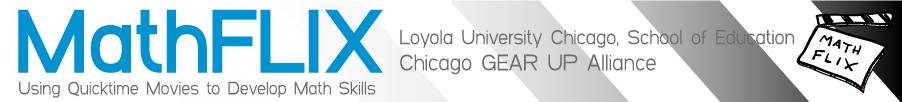### Content Index: G“G is for Googol: A Math Alphabet Book” - Guess & Check

• “G is for Googol: A Math Alphabet Book”
• Games
• coin
• decimal card game
• fractions
• magic tricks
• mean
• playing cards
• strategy
• tic-tac-toe
• Gears
• Geometry
• Graphing
• Graphing Calculators
• Graphing Equations
• coordinate geometry
• quadratic, cubic & absolute value
• truth tables
• y-intercept & slope
• Graphing Rate
• Graphs
• Guess & Check
• guess and check strategy